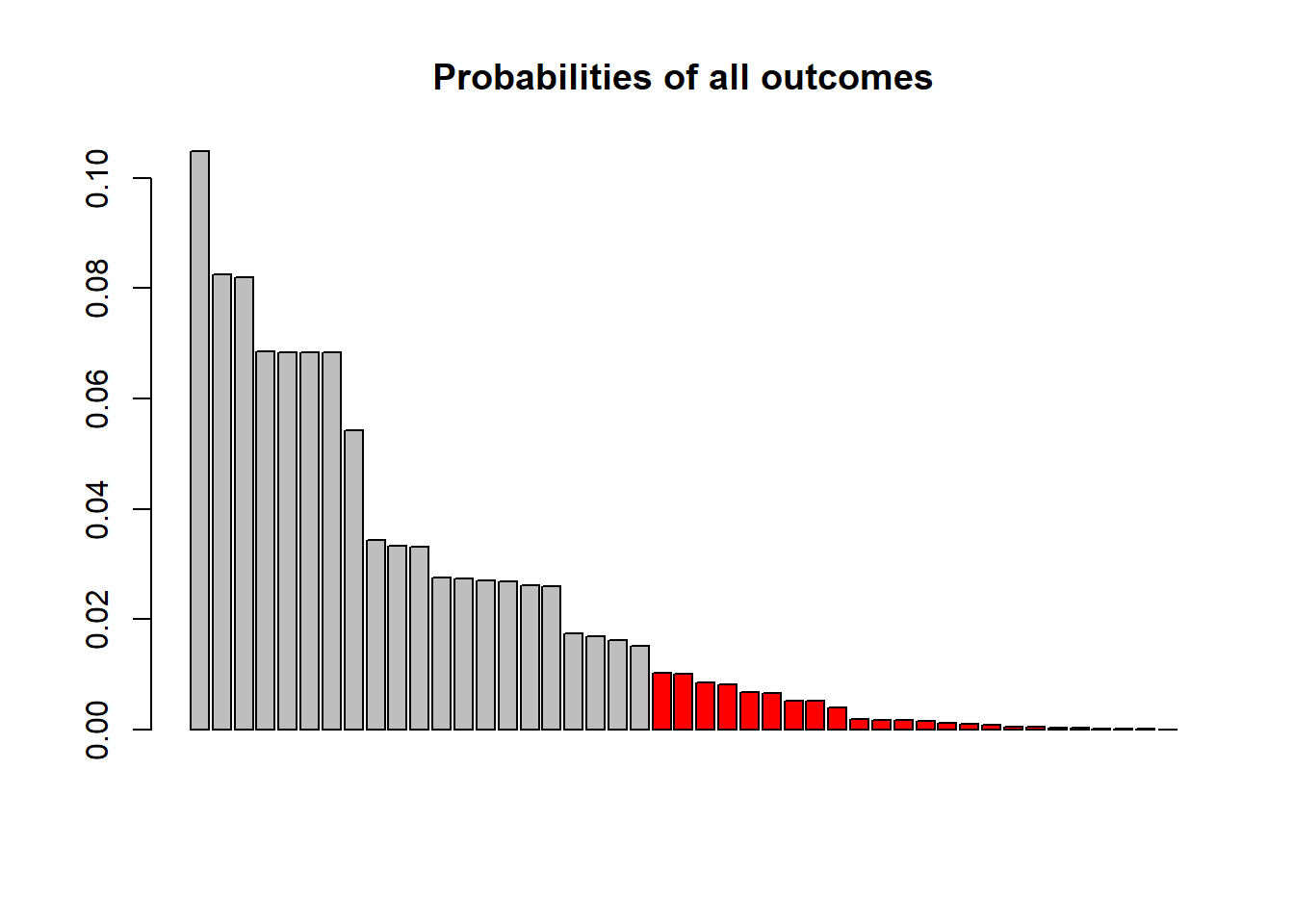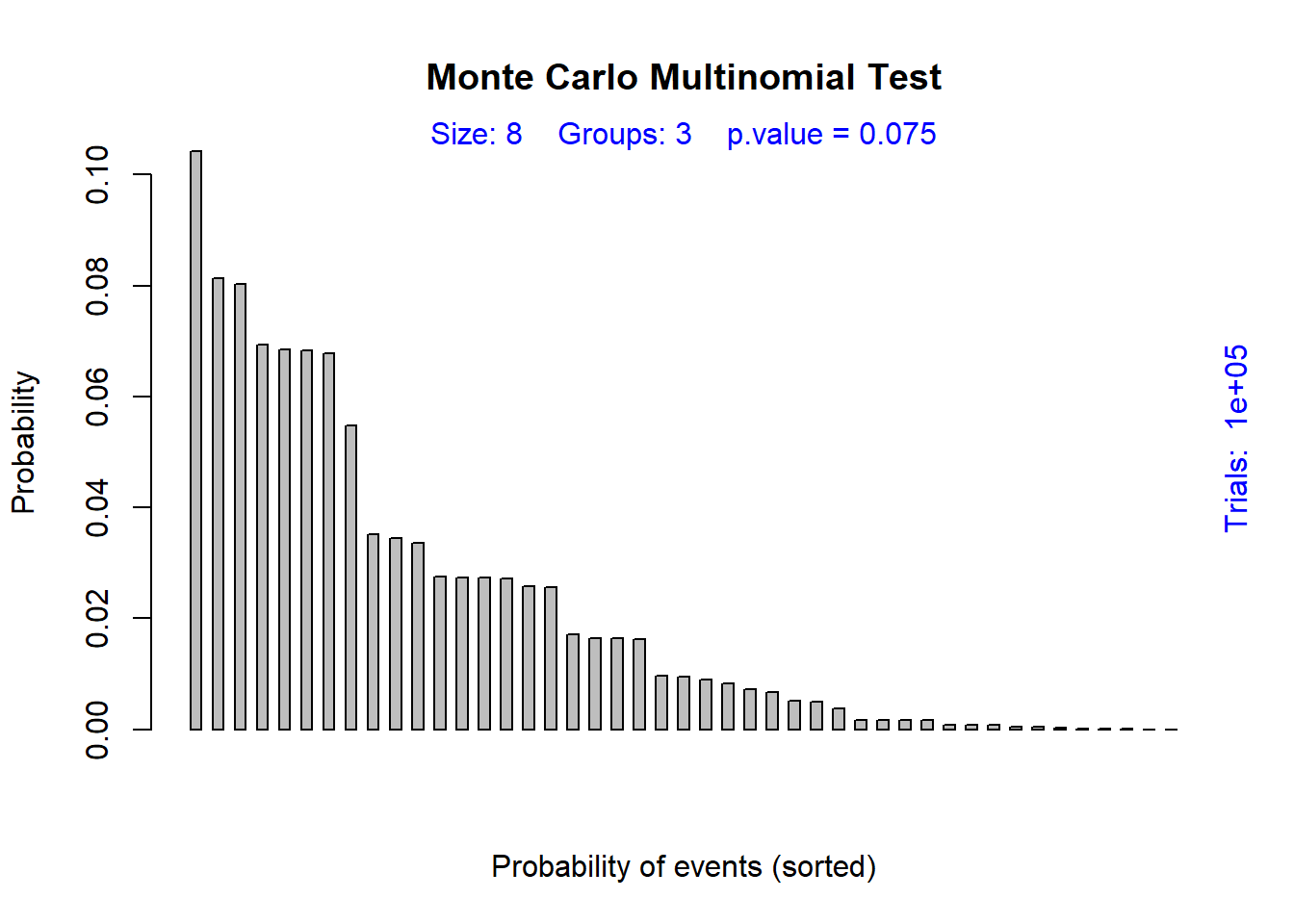### 1. Definition der Parameter

prob <- c(0.25, 0.5, 0.25)      # nullhypothesis
observed <- c(5, 2, 1)          # observed values
K = length(observed)            # number of categories
n = sum(observed)               # sample size
M = choose(n+K-1, K-1)          # number of possible tripletts
h0 <- c("red","red","brown","brown","brown","brown","green","green")  # 1:2:1
count_list = list()             # to store frequencies

### 2. Anzahl der Loops

• wie viele Beobachtungen ( = Ziehen von 8 Kugeln) wollen wir machen?
• es sollten viel mehr als die Anzahl der möglichen Resultate sein
• (wir fordern hier 100 Mal soviel)
loops = 100000
if(loops < 100*M) stop("Too few loops!\n")

### 3. Durchführen aller Beobachtungen

for (m in 1:loops) {
stichprobe <- sample(h0, size = 8, replace = TRUE)
x1 = sum(stichprobe == "red")
x2 = sum(stichprobe == "brown")
x3 = sum(stichprobe == "green")
triple = paste(x1,x2,x3, sep = "")
# cat(paste(triple ,"\n"))
if(is.null(count_list[[triple]])) {
count_list[[triple]] = 1
} else {
count_list[[triple]] = count_list[[triple]] + 1
}
}

### 4. p-Wert ausrechnen

• wir können bei wiederholtem Lauf des Programms nicht immer das gleiche Ergebnis erwarten
• … denn wir erzeugen ja jedesmal neue, zufällige Werte
name_observed = paste(observed, collapse = "")      # "521" (observation)
p.observed = count_list[[name_observed]] / loops    #  0.0112 = probability of the observation
p.observed = dmultinom(observed, n, prob)           #  or exact

# loop through all other triples and calculate their probabilities
p.value = 0
for (name in names(count_list)) {
p.name = count_list[[name]] / loops
cat(paste("triple:", name, "prob. =", p.name, "\n"))
if(p.name <= p.observed) p.value = p.value + p.name
}
## triple: 035 prob. = 0.00665
## triple: 251 prob. = 0.08245
## triple: 071 prob. = 0.01507
## triple: 233 prob. = 0.06843
## triple: 161 prob. = 0.05431
## triple: 341 prob. = 0.0684
## triple: 053 prob. = 0.02751
## triple: 242 prob. = 0.10488
## triple: 143 prob. = 0.0684
## triple: 332 prob. = 0.06849
## triple: 260 prob. = 0.02688
## triple: 152 prob. = 0.08193
## triple: 323 prob. = 0.03303
## triple: 224 prob. = 0.0259
## triple: 170 prob. = 0.01614
## triple: 134 prob. = 0.03433
## triple: 620 prob. = 0.00155
## triple: 431 prob. = 0.03337
## triple: 440 prob. = 0.01745
## triple: 521 prob. = 0.01008
## triple: 512 prob. = 0.00511
## triple: 062 prob. = 0.02705
## triple: 125 prob. = 0.01024
## triple: 422 prob. = 0.02609
## triple: 611 prob. = 0.00175
## triple: 710 prob. = 0.00026
## triple: 701 prob. = 1e-04
## triple: 350 prob. = 0.02727
## triple: 044 prob. = 0.01682
## triple: 305 prob. = 0.00078
## triple: 503 prob. = 0.00101
## triple: 404 prob. = 0.00108
## triple: 026 prob. = 0.00171
## triple: 215 prob. = 0.00518
## triple: 413 prob. = 0.00845
## triple: 080 prob. = 0.00401
## triple: 602 prob. = 0.00039
## triple: 530 prob. = 0.00669
## triple: 314 prob. = 0.00814
## triple: 107 prob. = 0.00011
## triple: 116 prob. = 0.00178
## triple: 017 prob. = 0.00023
## triple: 008 prob. = 3e-05
## triple: 206 prob. = 0.00045
## triple: 800 prob. = 2e-05
p.value  
##  0.0758

### 5. Visualisierung

• Das Säulendiagramm zeigt die Wahrscheinlichkeiten aller 45 möglichen Resultate des Versuchs.
• Alle Ereignisse, die eine kleinere Wahrscheinlichkeit als die Beobachtung haben, sind rot markiert.
• Die Summe dieser Wahrscheinlichkeiten ergibt den p-Wert des Signifikanztestes.
bar = sort(as.numeric(unlist(count_list)/loops), decreasing = TRUE)
color = rep("grey", length(bar))
color[bar <= p.observed] = "red"
barplot(bar, col = color, main = "Probabilities of all outcomes")### 6. Benutzung einer R-Bibliothek

• Die Bibliothek ‘EMT’ muss installiert sein
library(EMT)
## Warning: package 'EMT' was built under R version 3.5.2
res <- multinomial.test(observed, prob, useChisq = FALSE, MonteCarlo = TRUE, ntrial = 100000)
##
##  Monte Carlo Multinomial Test, distance measure: f
##
##     Events    fObs    p.value
##         45  0.0097      0.075
plotMultinom(res)Help me improve this site: uwe.menzel@matstat.org